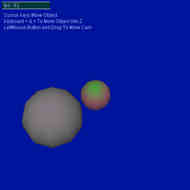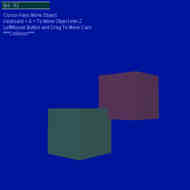Saturday August 15, 2020
 home | about | contact | DonationsThe Maths of 3D You can't have 3D without a little maths...

Collision Detection

by bkenwright@xbdev.net

Putting together various demo programs that show how you would go about testing for intersection tests with various common shapes, and how to determine the intersection point.  You usually determine if a collision has occured, then using the intersection point, determine how to react to the collision, as in games if a ball hit a wall you would want to know the hit point to you would work out where the ball would bounce back from :)Triangle Ray Collision Intersection DemoTriangle Line Collision Intersection Demo  Download Source CodeSphere Ray Collision Intersection DemoSphere Ray Collision Intersection Demo  Download Source CodeSphere - Sphere Collision Detection DemoSphere - Sphere Collision Detection Demo  Download Source CodeAABB - AABB Collision Detection DemoAABB - AABB Collision Detection Demo  Download Source CodeRay-Plane Intersection DemoRay-Plane Intersection Demo  Download Source CodeBaryCentric CoordinatesBaryCentric Coordinates is a way of representing a set of weighted values for each point.  The concept is used in numerous tests not just our triangle/point demo.  P = uA + vB + wC   P is our point, A,B,C our triangle and of course u,v,w are our Barycentric coordinates.   where u+v+w=1   The point is inside the triangle only if 0<= u,v,w <= 1,  or alternatively, if and only if 0<=v<=1.0f and 0<=w<=1 and  v+w<=1   Not that I say v+w<=1, as u=1-w-v   Which leads us to rearranging for what u is, and gives us:   P = A + v(B-A) + w(C-A) = (1-v-w)A + vB + wC   And we can solve for v and w.Quick View of the Various Intersection / Collision Detection Algorithms Source

Useful DirectX Librarys

• D3DXPlaneIntersectLine(..) - return the point on the plane or NULL if parallel

 Visitor: 9534626 { 229.27.38.75 } Copyright (c) 2002-2020 xbdev.net - All rights reserved. Designated articles, tutorials and software are the property of their respective owners.